• 概率密度的概念与性质 1.定义 2.性质 典型例题
概率密度的概念与性质 1.定义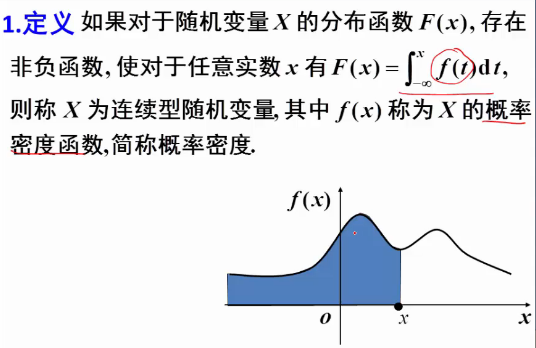2.性质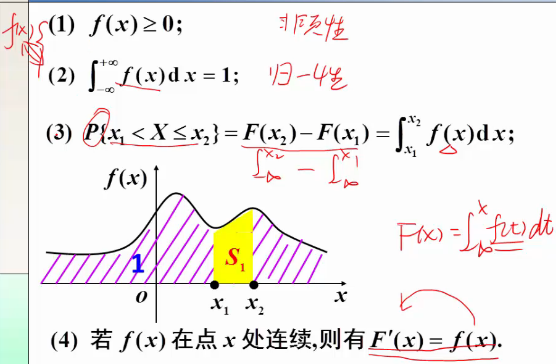典型例题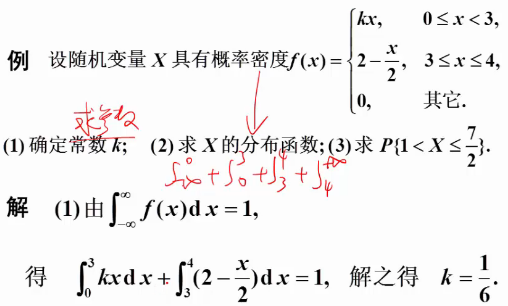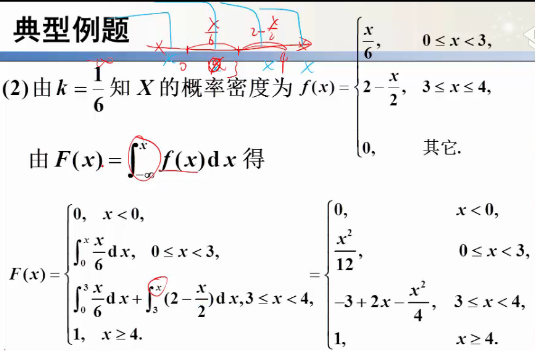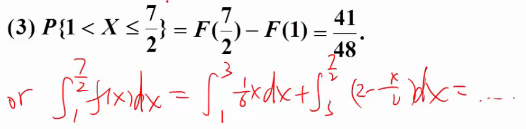展开全文• 总结的比较全的对与常见连续型分布函数，概率密度函数和特征函数的性质，并举出一些例题，是一个很好的参考资料
• 设X和Y的联合密度函数为： f(x,y)={20<x<∞,0<y<∞0其他区域f(x,y)=\left\{ \begin{array}{rcl} 2 && {0<x<\infty,0<y<\infty}\\ 0 && 其他区域 \end{array} \right.f(x,y)...
【例题】
设X和Y的联合密度函数为：
$f(x,y)=\left\{ \begin{array}{rcl} 2 && {0
计算（1）P{X>1,Y<1}  （2）P{X<Y}
类型题概述
这类给联合密度函数求概率的题实质上就是二重积分，被积函数是联合密度函数，积分区域是两个给出区域的交集：联合密度函数有意义的区域（即不为零的区域）与所求概率花括号中表示的区域（没看懂？没关系，结合例题秒懂！）
例题解析
来看具体例题：
（1） P{x>1, Y<1}
首先联合密度函数不为零的区域是：（为零的区域没有意义不考虑）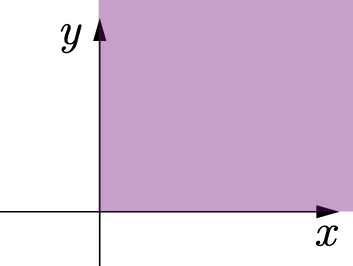然后第（1）题的所求概率区域是：x>1, Y<1（P{x>1, Y<1}） 即最后的积分区域D是两区域的交集——图中黄色部分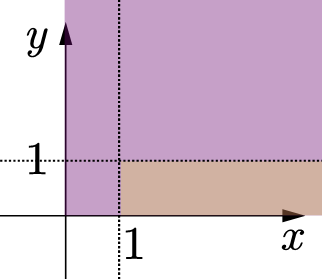对图中黄色区域D求联合密度的二重积分就可以了。（再次：联合密度为0的部分不考虑）
(1) P{x>1, Y<1}
$=\displaystyle\iint2e^{-x}e^{-2y}dxdy$
$=\displaystyle\int_1^{+\infty}dx\int_0^12e^{-x}e^{-2y}dy$
$=(1-e^{-2})\displaystyle\int_1^{+\infty}e^{-x}dx$
$=e^{-1} - e^{-3}$
（计算可能存在错误。你看看方法就行）
同理，（2）的联合密度函数没有变，所以它的被积函数与有效区域（紫色）也没有变。但求的概率不一样（P{x<y}）所以二者交叠的黄色区域如图所示：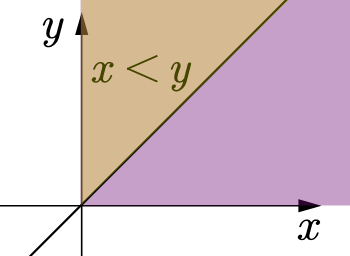P{x<y}
$=\displaystyle\iint2e^{-x}e^{-2y}dxdy$
$=\displaystyle\int_0^{+\infty}dx\int_0^x2e^{-x}e^{-2y}dy$
$=\displaystyle\int_1^{+\infty}e^{-x}-e^{-3x}dx$
$=e^{-1} - e^{-3}$
小结
再来看这句话：这类给联合密度函数求概率的题实质上就是二重积分，被积函数是联合密度函数，积分区域是两个给出区域的交集：联合密度函数有意义的区域（即不为零的区域）与所求概率花括号中表示的区域
是不是很easy！恭喜你学会了！
（啊今天居然有人问我概率论的题那我随便写一下吧。啊既然写了不如水一篇博客叭。顺带一提这里计算结果不一定是正确的因为博主我实在是口算笔算二重渣） 不重要大家掌握方法就好啦。希望对你有帮助！做错了请一定要告诉俺一声。。溜了溜了。。。


展开全文• 因为关于二维随机变量主题内容重要，难度大，例题多，最主要是积分区间的确定是难点，同时关联卷积概念，求二维函数Z=g(X,Y)型，用卷积公式求概率密度，卷积公式容易，积分区间难以确定，所以分成上中下三篇博客写。...
二维函数Z=g(X,Y)型，用卷积公式求概率密度，积分区域如何确定（上）

因为关于二维随机变量主题内容重要，难度大，例题多，最主要是积分区间的确定是难点，同时关联卷积概念，求二维函数Z=g(X,Y)型，用卷积公式求概率密度，卷积公式容易，积分区间难以确定，所以分成上中下三篇博客写。

一。问题的引入

有一大群人，令X和Y分别表示一个人的年龄和体重，Z表示该人的血压，并且已知Z与X,Y的关系为 Z=g(X,Y), 如何通过X,Y的分布确定Z的分布？二。公式

Fz(z)=P(Z⩽z)=∫∫g(x,y)⩽zf(x,y)dxdy${F}_{z}\left(z\right)=P\left(Z⩽z\right)=\int {\int }_{g\left(x,y\right)⩽z}f\left(x,y\right)dxdy$$F_{z}(z)=P(Z\leqslant z)=\int \int_{g(x,y)\leqslant z} f(x,y)dxdy$

特殊类型：Z=X+Y，怎样确定Z的分布？如何求Z的概率密度？
fz(z)=∫∞−∞f(x,z−x)dx=∫∞−∞f(z−y,y)dy${f}_{z}\left(z\right)={\int }_{-\mathrm{\infty }}^{\mathrm{\infty }}f\left(x,z-x\right)dx={\int }_{-\mathrm{\infty }}^{\mathrm{\infty }}f\left(z-y,y\right)dy$$f_{z}(z)=\int_{-\infty }^{\infty }f(x,z-x)dx = \int_{-\infty }^{\infty }f(z-y,y)dy$

当X与Y相互独立时，
就得到所谓的卷积公式$卷积公式$${\color{Red} {卷积公式}}$
fz(z)=fx∗fy=∫∞−∞fX(x)fY(z−x)dx=∫∞−∞fX(z−y)fY(y)dy${f}_{z}\left(z\right)={f}_{x}\ast {f}_{y}={\int }_{-\mathrm{\infty }}^{\mathrm{\infty }}{f}_{X}\left(x\right){f}_{Y}\left(z-x\right)dx={\int }_{-\mathrm{\infty }}^{\mathrm{\infty }}{f}_{X}\left(z-y\right){f}_{Y}\left(y\right)dy$$f_{z}(z)=f_{x}*f_{y}=\int_{-\infty }^{\infty }f_{X}(x)f_{Y}(z-x)dx = \int_{-\infty }^{\infty }f_{X}(z-y)f_{Y}(y)dy$
这就是所谓的卷积积分

三。已知f(x,y)，如何计算Z=X+Y型的概率密度fz(z)${f}_{z}\left(z\right)$$f_{z}(z)$ 及概率分布 Fz(z)${F}_{z}\left(z\right)$$F_{z}(z)$？

根据理解或者根据上面的公式，我们知道 fz(z)${f}_{z}\left(z\right)$$f_{z}(z)$ 是将f(x,y)求一次积分，Fz(z)${F}_{z}\left(z\right)$$F_{z}(z)$是求二次积分，难点问题在于如何确定积分区间？需要分成几个区间？$如何确定积分区间？需要分成几个区间？$${\color{Red} {如何确定积分区间？需要分成几个区间？}}$

对于Z=X+Y型的关系，假设对x求一次积分，得到fz(z)${f}_{z}\left(z\right)$$f_{z}(z)$
表示成

fz(z)=∫∞−∞f(x,z−x)dx${f}_{z}\left(z\right)={\int }_{-\mathrm{\infty }}^{\mathrm{\infty }}f\left(x,z-x\right)dx$$f_{z}(z)=\int_{-\infty }^{\infty }f(x,z-x)dx$

，那么我们要画出一个 x–z的坐标，确定积分区间

1）积分区间的左右两边，由x的上下区间决定
假设 x的区间在[a,b]之间, a⩽x⩽b$a⩽x⩽b$$a\leqslant x\leqslant b$
那么积分的左右边界就是a到b
2）根据关系式
z=x+y， 由于坐标系是x–z的关系，那么y就是变常量
z的最小值：zmin=x+ymin${z}_{min}=x+{y}_{min}$$z_{min}=x+y_{min}$
z的最大值：zmax=x+ymax${z}_{max}=x+{y}_{max}$$z_{max}=x+y_{max}$
积分的上下边界就是 zmin${z}_{min}$$z_{min}$到 zmax${z}_{max}$$z_{max}$

因为我们讨论的f_{z}(z)是按照x积分：
fz(z)=∫∞−∞f(x,z−x)dx${f}_{z}\left(z\right)={\int }_{-\mathrm{\infty }}^{\mathrm{\infty }}f\left(x,z-x\right)dx$$f_{z}(z)=\int_{-\infty }^{\infty }f(x,z-x)dx$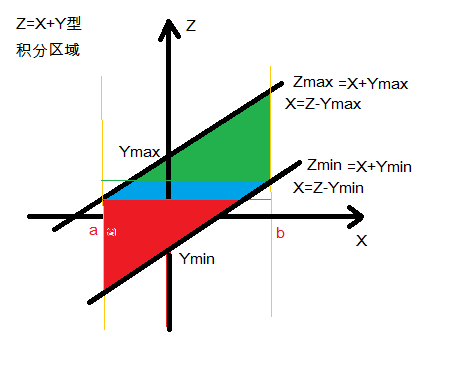所以按照x积分，积分区间就要分成三段：红色区间，蓝色区间，绿色区间

1)红色区间$红色区间$${\color{Red} {红色区间}}$，
Xmin+Ymin⩽z<Xmin+Ymax${X}_{min}+{Y}_{min}⩽z<{X}_{min}+{Y}_{max}$$X_{min}+Y_{min} \leqslant z
x积分区间= a 到 Z-Ymin
∫z−yminadx${\int }_{a}^{z-{y}_{min}}dx$$\int_{a}^{z-y_{min}}dx$

2)蓝色区间$蓝色区间$${\color{Blue} {蓝色区间}}$，
Xmin+Ymax⩽z<Xmax+Ymin${X}_{min}+{Y}_{max}⩽z<{X}_{max}+{Y}_{min}$$X_{min}+Y_{max} \leqslant z
x积分区间= Z-Ymax 到 Z-Ymin
∫z−yminz−ymaxdx${\int }_{z-{y}_{max}}^{z-{y}_{min}}dx$$\int_{z-y_{max}}^{z-y_{min}}dx$

3)绿色区间$绿色区间$${\color{Green} {绿色区间}}$，
Xmax+Ymin⩽z<Xmax+Ymax${X}_{max}+{Y}_{min}⩽z<{X}_{max}+{Y}_{max}$$X_{max}+Y_{min} \leqslant z

x积分区间= Z-Ymax 到  1
∫bz−ymaxdx${\int }_{z-{y}_{max}}^{b}dx$$\int_{z-y_{max}}^{b}dx$

当x的a,b左右对称时，中间蓝色区间没有，只有两个积分区间：
红色区间$红色区间$${\color{Red} {红色区间}}$和 绿色区间$绿色区间$${\color{Green} {绿色区间}}$

=========================
【例一】设(X,Y)的联合密度函数为
f(x,y)={ e−y,0⩽x⩽1,y⩾0, 0,othersf(x,y)= \begin{cases}
& \text{ } e^{-y}, 0\leqslant x\leqslant 1, y\geqslant 0, \\
& \text{ } 0,    others
\end{cases}
(1)问X,Y是否独立？
(2)求Z=2X+Y的密度函数fz(z)${f}_{z}\left(z\right)$$f_{z}(z)$和分布函数Fz(z)${F}_{z}\left(z\right)$$F_{z}(z)$
(3)求P{Z>3}

【解】
(1) 问X,Y是否独立？
X,Y独立的条件 f(x,y)=fx(x)∗fy(y)$f\left(x,y\right)={f}_{x}\left(x\right)\ast {f}_{y}\left(y\right)$$f(x,y)=f_{x}(x)*f_{y}(y)$
fX(x)=∫∞−∞f(x,y)dy${f}_{X}\left(x\right)={\int }_{-\mathrm{\infty }}^{\mathrm{\infty }}f\left(x,y\right)dy$$f_{X}(x)=\int_{-\infty }^{\infty}f(x,y)dy$
fX(x)=∫∞0e−ydy=−e−y|∞0=e−y|0∞=1${f}_{X}\left(x\right)={\int }_{0}^{\mathrm{\infty }}{e}^{-y}dy=-{e}^{-y}{|}_{0}^{\mathrm{\infty }}={e}^{-y}{|}_{\mathrm{\infty }}^{0}=1$$f_{X}(x)=\int_{0 }^{\infty}e^{-y}dy=-e^{-y}|^\infty_{0}=e^{-y}|^0_{\infty}=1$

fY(y)=∫∞−∞f(x,y)dx${f}_{Y}\left(y\right)={\int }_{-\mathrm{\infty }}^{\mathrm{\infty }}f\left(x,y\right)dx$$f_{Y}(y)=\int_{-\infty }^{\infty}f(x,y)dx$
fY(y)=∫∞0e−ydx=∫10e−ydx=e−y∗(x)|10=e−y${f}_{Y}\left(y\right)={\int }_{0}^{\mathrm{\infty }}{e}^{-y}dx={\int }_{0}^{1}{e}^{-y}dx={e}^{-y}\ast \left(x\right){|}_{0}^{1}={e}^{-y}$$f_{Y}(y)=\int_{0 }^{\infty}e^{-y}dx= \int_{0 }^{1}e^{-y}dx=e^{-y}*(x)|^1_{0}=e^{-y}$
所以 f(x,y)=fX(x)∗fY(y)$f\left(x,y\right)={f}_{X}\left(x\right)\ast {f}_{Y}\left(y\right)$$f(x,y)=f_{X}(x)*f_{Y}(y)$

(2)求Z=2X+Y的密度函数fz(z)${f}_{z}\left(z\right)$$f_{z}(z)$和分布函数Fz(z)${F}_{z}\left(z\right)$$F_{z}(z)$

(2.1)先求密度函数fz(z)${f}_{z}\left(z\right)$$f_{z}(z)$

Z=g(X,Y)=2X+Y

求fz(z)${f}_{z}\left(z\right)$$f_{z}(z)$可以利用卷积公式
fZ(z)=∫∞−∞f(x,z−x)dx${f}_{Z}\left(z\right)={\int }_{-\mathrm{\infty }}^{\mathrm{\infty }}f\left(x,z-x\right)dx$$f_{Z}(z)=\int_{-\infty}^{\infty}f(x,z-x)dx$

画一个 x-z 的坐标系

Z方向下限：
Zmin=g(X,Ymin)=2X+Ymin${Z}_{min}=g\left(X,{Y}_{min}\right)=2X+{Y}_{min}$$Z_{min}=g(X,Y_{min})=2X+Y_{min}$ = 2X+0

Z方向上限：
Zmin=g(X,Ymin)=2X+Ymax=2X+∞=∞${Z}_{min}=g\left(X,{Y}_{min}\right)=2X+{Y}_{max}=2X+\mathrm{\infty }=\mathrm{\infty }$$Z_{min}=g(X,Y_{min})=2X+Y_{max} = 2X+\infty =\infty$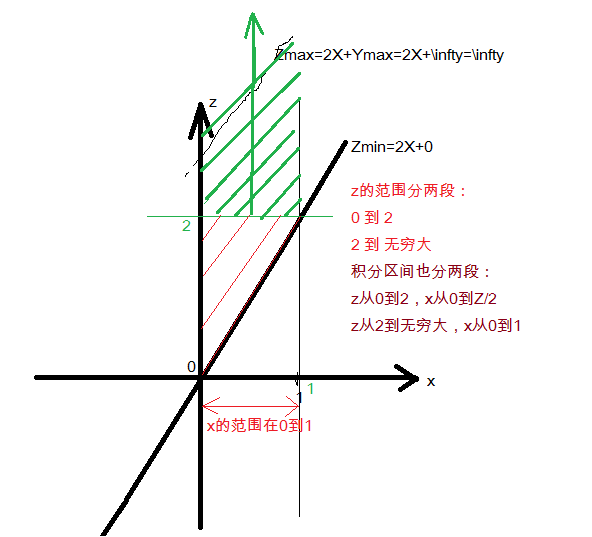所以，对公式 fZ(z)=∫∞−∞f(x,z−x)dx${f}_{Z}\left(z\right)={\int }_{-\mathrm{\infty }}^{\mathrm{\infty }}f\left(x,z-x\right)dx$$f_{Z}(z)=\int_{-\infty}^{\infty}f(x,z-x)dx$

当 0⩽z<2:0⩽x<z2$0⩽z<2:0⩽x<\frac{z}{2}$$0\leqslant z < 2 : 0\leqslant x < \frac{z}{2}$
fZ(z)=∫z20f(x,z−x)dx=∫z20e−(z−2x)dx${f}_{Z}\left(z\right)={\int }_{0}^{\frac{z}{2}}f\left(x,z-x\right)dx={\int }_{0}^{\frac{z}{2}}{e}^{-\left(z-2x\right)}dx$$f_{Z}(z)=\int_{0}^{\frac{z}{2}}f(x,z-x)dx = \int_{0}^{\frac{z}{2}} e^{-(z-2x)}dx$
∫z20e2x−zdx(设t=2x−z,dt=2dx)${\int }_{0}^{\frac{z}{2}}{e}^{2x-z}dx\left(设t=2x-z,dt=2dx\right)$$\int_{0}^{\frac{z}{2}}e^{2x-z}dx (设t=2x-z, dt=2dx)$
=12e2x−z|z20$\frac{1}{2}{e}^{2x-z}{|}_{0}^{\frac{z}{2}}$$\frac{1}{2} e^{2x-z}|_0^\frac{z}{2}$
=12(1−e−z)$\frac{1}{2}\left(1-{e}^{-z}\right)$$\frac{1}{2}(1-e^{-z})$

当 2⩽z<∞:0⩽x⩽1$2⩽z<\mathrm{\infty }:0⩽x⩽1$$2\leqslant z < \infty : 0\leqslant x \leqslant 1$
fZ(z)=∫10f(x,z−x)dx=∫10e−(z−2x)dx${f}_{Z}\left(z\right)={\int }_{0}^{1}f\left(x,z-x\right)dx={\int }_{0}^{1}{e}^{-\left(z-2x\right)}dx$$f_{Z}(z)=\int_{0}^{1}f(x,z-x)dx = \int_{0}^{1} e^{-(z-2x)}dx$
∫10e2x−zdx(设t=2x−z,dt=2dx)${\int }_{0}^{1}{e}^{2x-z}dx\left(设t=2x-z,dt=2dx\right)$$\int_{0}^{1}e^{2x-z}dx (设t=2x-z, dt=2dx)$
=12e2x−z|10$\frac{1}{2}{e}^{2x-z}{|}_{0}^{1}$$\frac{1}{2} e^{2x-z}|_0^1$
=12(e2−1)e−z$\frac{1}{2}\left({e}^{2}-1\right){e}^{-z}$$\frac{1}{2}(e^2-1)e^{-z}$

所以 fZ(z)=⎧⎩⎨⎪⎪ 0,z<0, 12(1−e−z),0⩽z⩽2, 12(e2−1)e−z,z>2f_{Z}(z)=
\begin{cases}
& \text{ } 0,z<0, \\
& \text{ } \frac{1}{2}(1-e^{-z}), 0\leqslant z\leqslant 2, \\
& \text{ } \frac{1}{2}(e^2-1)e^{-z}, z> 2
\end{cases}

(2.2) 求分布函数Fz(z)${F}_{z}\left(z\right)$$F_{z}(z)$

由分布函数Fz(z)${F}_{z}\left(z\right)$$F_{z}(z)$的定义可以知道，就是对z再积分
Fz(z)${F}_{z}\left(z\right)$$F_{z}(z)$与相应的概率密度函数fZ(z)${f}_{Z}\left(z\right)$$f_{Z}(z)$的积分区间的关系是怎样呢？
概率密度函数fZ(z)${f}_{Z}\left(z\right)$$f_{Z}(z)$是对横坐标x积分，分布函数Fz(z)${F}_{z}\left(z\right)$$F_{z}(z)$是对纵坐标z进行积分。通过z进行分区段，Fz(z)${F}_{z}\left(z\right)$$F_{z}(z)$与fz(z)${f}_{z}\left(z\right)$$f_{z}(z)$是一样的，但是Fz(z)${F}_{z}\left(z\right)$$F_{z}(z)$是把fz(z)${f}_{z}\left(z\right)$$f_{z}(z)$再次对z求积分，z的上下限的取值与z的分段不完全一样。

对z积分上下限取值原则：z的取值一直是从小到大方向，下限固定，上限活动，上限就是z$对z积分上下限取值原则：z的取值一直是从小到大方向，下限固定，上限活动，上限就是z$${\color{Red} {对z积分上下限取值原则：z的取值一直是从小到大方向，下限固定，上限活动，上限就是z}}$

FZ(z)=∫∞−∞fZ(z)dz${F}_{Z}\left(z\right)={\int }_{-\mathrm{\infty }}^{\mathrm{\infty }}{f}_{Z}\left(z\right)dz$$F_{Z}(z)=\int_{-\infty}^{\infty}f_{Z}(z)dz$
根据fZ(z)${f}_{Z}\left(z\right)$$f_{Z}(z)$的分段，分段再积分
所以，
z<0 时，F_{Z}(z)=0
当 0⩽z<2$0⩽z<2$$0\leqslant z < 2$ : z的积分区间：下限固定，下限是0，上限活动，上限是z$下限固定，下限是0，上限活动，上限是z$${\color{Red} {下限固定，下限是0，上限活动，上限是z}}$，所以就是 在0⩽z<z$0⩽z$0\leqslant z < z$
FZ(z)=∫z0fZ(z)dz=∫z012(1−e−z)dz${F}_{Z}\left(z\right)={\int }_{0}^{z}{f}_{Z}\left(z\right)dz={\int }_{0}^{z}\frac{1}{2}\left(1-{e}^{-z}\right)dz$$F_{Z}(z)=\int_{0}^{z}f_{Z}(z)dz = \int_{0}^{z} \frac{1}{2}(1-e^{-z})dz$
=12(z+e−z)|z0=12(z−1+e−z)$\frac{1}{2}\left(z+{e}^{-z}\right){|}_{0}^{z}=\frac{1}{2}\left(z-1+{e}^{-z}\right)$$\frac{1}{2}(z+e^{-z})|_{0}^{z}=\frac{1}{2}(z-1+e^{-z})$

当 2⩽z<∞$2⩽z<\mathrm{\infty }$$2\leqslant z < \infty$ :  注意分布函数与密度函数的区别，分布函数是对z的累加，要把前面的所有区间全部累加起来$要把前面的所有区间全部累加起来$${\color{Red} {要把前面的所有区间全部累加起来 }}$
当 2⩽z<∞$2⩽z<\mathrm{\infty }$$2\leqslant z < \infty$：z的积分区间为前面一段区间： 0到2，再加上当前区间，下限固定，下限就是2，上限活动，上限就是z$下限固定，下限就是2，上限活动，上限就是z$${\color{Red} {下限固定，下限就是2，上限活动，上限就是z}}$

FZ(z)=∫20fZ(z)dz+∫z2fZ(z)dz=${F}_{Z}\left(z\right)={\int }_{0}^{2}{f}_{Z}\left(z\right)dz+{\int }_{2}^{z}{f}_{Z}\left(z\right)dz=$$F_{Z}(z)=\int_{0}^{2}f_{Z}(z)dz + \int_{2}^{z}f_{Z}(z)dz=$
=∫2012(1−e−z)dz+∫z212(e2−1)e−zdz$={\int }_{0}^{2}\frac{1}{2}\left(1-{e}^{-z}\right)dz+{\int }_{2}^{z}\frac{1}{2}\left({e}^{2}-1\right){e}^{-z}dz$$={\color{Red} {\int_{0}^{2}\frac{1}{2}(1-e^{-z}) dz}}+ \int_{2}^{z}\frac{1}{2}(e^2-1)e^{-z}dz$
=1+12(1−e2)e−z$=1+\frac{1}{2}\left(1-{e}^{2}\right){e}^{-z}$$=1+\frac{1}{2}(1-e^2)e^{-z}$

所以FZ(z)=⎧⎩⎨⎪⎪ 0,z<0 12(z−1+e−z),0⩽z<2 1+12(1−e2)e−z,z⩾2F_{Z}(z)=
\begin{cases}
& \text{  } 0, z<0 \\
& \text{  } \frac{1}{2}(z-1+e^{-z}),0\leqslant z<2 \\
& \text{  } 1+\frac{1}{2}(1-e^2)e^{-z}, z\geqslant 2
\end{cases}

(3)求P{Z>3}

求P(f(Z))总是跟分布函数FZ(z)${F}_{Z}\left(z\right)$$F_{Z}(z)$联系在一起的。根据概率分布函数的定义 FZ(z)${F}_{Z}\left(z\right)$$F_{Z}(z)$指的是从−∞$-\mathrm{\infty }$$-\infty$到当前z的累加，运算值和查表值都只是 −∞$-\mathrm{\infty }$$-\infty$到某个当前z值得积分，即，积分的结果表示的是 P(Z⩽z)$P\left(Z⩽z\right)$$P(Z\leqslant z)$的值

所以
P(Z>3)=1−P(Z⩽3)=1−FZ(z)(z=3)$P\left(Z>3\right)=1-P\left(Z⩽3\right)=1-{F}_{Z}\left(z\right)\left(z=3\right)$$P(Z>3)=1-P(Z\leqslant 3)=1-F_{Z}(z)(z=3)$

根据上面的积分结果
FZ(3)=(1+12(1−e2)e−z)|z=3${F}_{Z}\left(3\right)=\left(1+\frac{1}{2}\left(1-{e}^{2}\right){e}^{-z}\right){|}_{z=3}$$F_{Z}(3) = ( 1+\frac{1}{2}(1-e^2)e^{-z})|_{z=3}$

P(Z>3)=1−(1+12(1−e2))e−3$P\left(Z>3\right)=1-\left(1+\frac{1}{2}\left(1-{e}^{2}\right)\right){e}^{-3}$$P(Z>3) =1-(1+\frac{1}{2}(1-e^2))e^{-3}$
=12(e2−1)e−3≈0.1591$=\frac{1}{2}\left({e}^{2}-1\right){e}^{-3}\approx 0.1591$$= \frac{1}{2}(e^2-1)e^{-3} \approx 0.1591$

参考书目：

张天德，叶宏 《星火燎原·概率论与数理统计辅导及习题精解》（浙大·第4版）第三章
展开全文卷积公式
• 因为关于二维随机变量主题内容重要，难度大，例题多，最主要是积分区间...求二维函数Z=g(X,Y)型，用卷积公式求概率密度，积分区域如何确定（中） #### ======= 【例二】 设二维随机变量(X,Y)的概率密度为f(x...
因为关于二维随机变量主题内容重要，难度大，例题多，最主要是积分区间的确定是难点，同时关联卷积概念，卷积公式容易，积分区间难以确定，因为书上的例题都没有详细解释积分区间如何确定，所以分成上中下三篇博客写。
接本主题（上）。

求二维函数Z=g(X,Y)型，用卷积公式求概率密度，积分区域如何确定（中）

 ####  =======


【例二】

设二维随机变量(X,Y)的概率密度为f(x,y)={ 2−x−y,0<x<1,0<y<1, 0,othersf(x,y)= \begin{cases}
& \text{ } 2-x-y,   0< x< 1, 0< y< 1, \\
& \text{ } 0,    others
\end{cases}
(1)求P{X>2Y}
(2)求Z=X+Y的密度函数fz(z)${f}_{z}\left(z\right)$$f_{z}(z)$

【解】

(1)求P{X>2Y}

一般的解法：P(f(X,Y))总是通过概率分布FZ(z)${F}_{Z}\left(z\right)$$F_{Z}(z)$得来的，而 FZ(z)${F}_{Z}\left(z\right)$$F_{Z}(z)$又是通过概率密度积分得出来的。概率密度 fZ(z)=∫∞−∞f(x,z−x)dx${f}_{Z}\left(z\right)={\int }_{-\mathrm{\infty }}^{\mathrm{\infty }}f\left(x,z-x\right)dx$$f_{Z}(z) = \int_{-\infty}^{\infty}f(x,z-x)dx$
要求P{X>2Y}，
设Z=X-2Y, 求P(X>2Y)就是求P(Z>0)=1−FZ(z⩽0)$P\left(Z>0\right)=1-{F}_{Z}\left(z⩽0\right)$$P(Z>0) = 1-F_{Z}(z\leqslant 0)$
先求概率密度 fZ(z)=∫∞−∞f(x,z−x)dx${f}_{Z}\left(z\right)={\int }_{-\mathrm{\infty }}^{\mathrm{\infty }}f\left(x,z-x\right)dx$$f_{Z}(z) = \int_{-\infty}^{\infty}f(x,z-x)dx$

如上所述，如果按照先求概率密度的方法来算一个具体的特定的概率，求概率密度再求分布函数，会比较麻烦，对于一个特定的概率，可以通过画图图解法，看看积分区域在哪里，直接求dxdy的双重积分，也许对于特定概率来说更加方便。

对本题P(X>2Y) ，直接画一个x,y的坐标图，很容易看出来X>2Y的区域在哪里，再结合题目规定的区间  0< x< 1, 0< y< 1, 很容易算出，dxdy的双重积分。
在x,y的坐标系上，直接画一条Y=12X$Y=\frac{1}{2}X$$Y=\frac{1}{2}X$的直线，题目要求X>2Y，那么就是Y<\frac{1}{2}X，很容易看出来区域在哪里。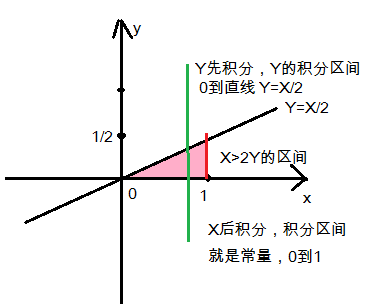图中粉红色的三角形区域就是X>2Y的区间，其中x限制在0到1，y也限制在0到1
先对Y积分，Y的积分区间就是0到直线Y=X/2；后对X积分，X的积分区间就是常量：0到1
P(X>2Y)=∫∫X>2Yf(x,y)dxdy$P\left(X>2Y\right)=\int {\int }_{X>2Y}f\left(x,y\right)dxdy$$P(X>2Y)=\int \int _{X>2Y} f(x,y)dxdy$
=∫10dx∫x20f(x,y)dy$={\int }_{0}^{1}dx{\int }_{0}^{\frac{x}{2}}f\left(x,y\right)dy$$=\int_{0}^{1}dx\int_{0}^{\frac{x}{2}}f(x,y)dy$
=∫10dx∫x20(2−x−y)dy=∫10dx((2−x)y−y22)|2x0$={\int }_{0}^{1}dx{\int }_{0}^{\frac{x}{2}}\left(2-x-y\right)dy={\int }_{0}^{1}dx\left(\left(2-x\right)y-\frac{{y}^{2}}{2}\right){|}_{0}^{\frac{2}{x}}$$=\int_{0}^{1}dx\int_{0}^{\frac{x}{2}}(2-x-y)dy = \int_{0}^{1}dx ((2-x)y-\frac{y^2}{2})|^\frac{2}{x}_0$
=∫10(x−58x2)dx=(x22−58∗x33)|10=12−58∗13=724$={\int }_{0}^{1}\left(x-\frac{5}{8}{x}^{2}\right)dx=\left(\frac{{x}^{2}}{2}-\frac{5}{8}\ast \frac{{x}^{3}}{3}\right){|}_{0}^{1}=\frac{1}{2}-\frac{5}{8}\ast \frac{1}{3}=\frac{7}{24}$$=\int_{0}^{1}(x-\frac{5}{8}x^2)dx = (\frac{x^2}{2}-\frac{5}{8}*\frac{x^3}{3})|^1_0=\frac{1}{2}-\frac{5}{8}*\frac{1}{3}=\frac{7}{24}$

(2)求Z=X+Y的密度函数fz(z)${f}_{z}\left(z\right)$$f_{z}(z)$

画出x-z坐标，算出两条直线范围，
Zmin=X+Ymin =X+0
Zmax=X+Ymax =X+1
加上X的0到1区间范围，就构成积分区间。如图，积分区间就是红色三角块和蓝色三角块。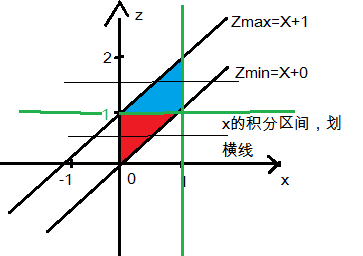fZ(z)=∫∞−∞f(x,z−x)dx${f}_{Z}\left(z\right)={\int }_{-\mathrm{\infty }}^{\mathrm{\infty }}f\left(x,z-x\right)dx$$f_{Z}(z) = \int_{-\infty}^{\infty}f(x,z-x)dx$
因此，积分区间按照z轴从下到上分，

当z<0,  由于z不在Zmin到Zmax的范围内，所以 fZ(z)=0${f}_{Z}\left(z\right)=0$$f_{Z}(z)=0$

当0⩽z<1$0⩽z<1$$0\leqslant z<1$,  对x先积分，x的积分区间为红色区块，x的边界为0到Zmin直线，所以积分区间就是0到z
fZ(z)=∫z0(2−x−(z−x))dx=∫z0(2−z)dx=(2−z)x|z0=(2−z)∗z${f}_{Z}\left(z\right)={\int }_{0}^{z}\left(2-x-\left(z-x\right)\right)dx={\int }_{0}^{z}\left(2-z\right)dx=\left(2-z\right)x{|}_{0}^{z}=\left(2-z\right)\ast z$$f_{Z}(z)=\int_{0}^{z}(2-x-(z-x))dx=\int_{0}^{z}(2-z)dx=(2-z)x|^z_0=(2-z)*z$

当1⩽z<2$1⩽z<2$$1\leqslant z<2$,  对x先积分，x的积分区间为蓝色区块，x的边界为Zmax直线到1，所以积分区间就是z-1到1
fZ(z)=∫1z−1(2−x−(z−x))dx=∫1z−1(2−z)dx=(2−z)x|1z−1=(2−z)2${f}_{Z}\left(z\right)={\int }_{z-1}^{1}\left(2-x-\left(z-x\right)\right)dx={\int }_{z-1}^{1}\left(2-z\right)dx=\left(2-z\right)x{|}_{z-1}^{1}=\left(2-z{\right)}^{2}$$f_{Z}(z)=\int_{z-1}^{1}(2-x-(z-x))dx=\int_{z-1}^{1}(2-z)dx=(2-z)x|^1_{z-1}=(2-z)^2$

所以，概率密度fZ(z)=⎧⎩⎨ z(2−z),0⩽z<1 (2−z)2,1⩽z<2 0,elsef_{Z}(z) =
\begin{cases}
& \text{ } z(2-z),0\leqslant z<1 \\
& \text{ } (2-z)^2,1\leqslant z<2 \\
& \text{ } 0, else
\end{cases}

####  =====


【例三】

假设一电路装有3个同种电气元件，其工作状态相互独立，且无故障工作时间都服从参数为λ>0$\lambda >0$$\lambda > 0$的指数分布，当3个元件都无故障时，电路正常工作，否则整个电路不能正常工作，试求电路正常工作的时间T的概率分布。

【解】
分布函数的物理含义：F(x)表示在x范围内（X⩽x)发生事件的概率。$F\left(x\right)表示在x范围内（X⩽x\right)发生事件的概率。$${\color{Red}{F(x)表示在x范围内（X\leqslant x)发生事件的概率。}}$

指数分布函数的含义：F(t)=1−e−λt，F(t)表示x时间前发生故障的概率。$F\left(t\right)=1-{e}^{-\lambda t}，F\left(t\right)表示x时间前发生故障的概率。$${\color{Red}{F(t)=1-e^{-\lambda t}，F(t)表示x时间前发生故障的概率。}}$

指数(概率密度)函数的意义：f(t)=e−λt，表示t时间内不发生故障的概率。$f\left(t\right)={e}^{-\lambda t}，表示t时间内不发生故障的概率。$${\color{Red}{f(t)=e^{-\lambda t}，表示t时间内不发生故障的概率。}}$

[分析]
关于指数分布，回顾一下博客离散型随机变量，二项分布，泊松分布，指数分布，几何分布（概统2.知识）
泊松分布是求某个时间t内，事件发生k次的概率
P{ X=k }=(λt)kk!∗e−λt$\frac{\left(\lambda t{\right)}^{k}}{k!}\ast {e}^{-\lambda t}$$\frac{ ({\lambda t})^k} {k!}*e^{-\lambda t}$
“所谓指数分布就是求事件发生的时间间隔”
P(X=0,N(t))=(λt)00!∗e−λt=e−λt$P\left(X=0,N\left(t\right)\right)=\frac{\left(\lambda t{\right)}^{0}}{0!}\ast {e}^{-\lambda t}={e}^{-\lambda t}$$P(X=0,N(t)) =\frac{(\lambda t)^0}{0!}*e^{-\lambda t}=e^{-\lambda t}$
“在t时间内出现一个以上的概率”
P{X>0,N(t)}=1−e−λt$P\left\{X>0,N\left(t\right)\right\}=1-{e}^{-\lambda t}$$P\{ X>0,N(t) \}=1-e^{-\lambda t}$

指数分布，
概率密度函数为f(x)=λe−λx$f\left(x\right)=\lambda {e}^{-\lambda x}$$f(x)=\lambda e^{-\lambda x}$
分布函数为F(x)=1−e−λx$F\left(x\right)=1-{e}^{-\lambda x}$$F(x)=1-e^{-\lambda x}$
简写 X∼E(λ)$X\sim E\left(\lambda \right)$$X \sim E(\lambda)$
参数λ$\lambda$$\lambda$为单位时间内事件发生的次数，即（电子元器件损坏的次数）。
所以电子元器件的平均寿命就是 指数分布的期望值 EX=1λ$EX=\frac{1}{\lambda }$$EX=\frac{1}{\lambda}$
假设某电子元器件的平均寿命为1000小时，那么EX=1000,λ=0.001$EX=1000,\lambda =0.001$$EX=1000, \lambda=0.001$,
某个电子元器件在1000小时内损坏的概率就是分布函数F(x<1000)
F(x<1000)=1−e−λx=1−1eλx$F\left(x<1000\right)=1-{e}^{-\lambda x}=1-\frac{1}{{e}^{\lambda x}}$$F(x<1000)=1-e^{-\lambda x} =1-\frac{1}{e^{\lambda x}}$
=1−1e0.001∗1000=1−1e$=1-\frac{1}{{e}^{0.001\ast 1000}}=1-\frac{1}{e}$$= 1 - \frac{1}{e^{0.001*1000}} = 1-\frac{1}{e}$

分布函数的物理含义：F(x)表示在x范围内（X⩽x)发生事件的概率。$F\left(x\right)表示在x范围内（X⩽x\right)发生事件的概率。$${\color{Red}{F(x)表示在x范围内（X\leqslant x)发生事件的概率。}}$

指数分布函数的含义：F(t)=1−e−λt$F\left(t\right)=1-{e}^{-\lambda t}$$F(t)=1-e^{-\lambda t}$，F(t)表示x时间前发生故障的概率。$F\left(t\right)表示x时间前发生故障的概率。$${\color{Red}{F(t)表示x时间前发生故障的概率。}}$

指数(概率密度)函数的意义：f(t)=e−λt$f\left(t\right)={e}^{-\lambda t}$$f(t)=e^{-\lambda t}$，表示t时间内不发生故障的概率。$表示t时间内不发生故障的概率。$${\color{Red}{表示t时间内不发生故障的概率。}}$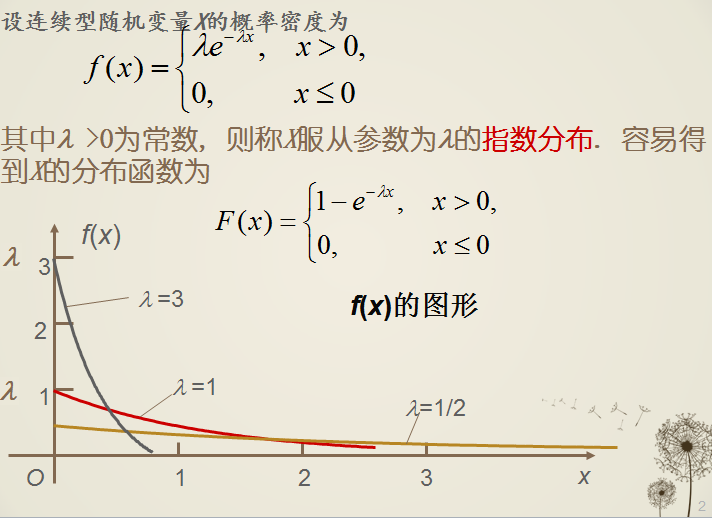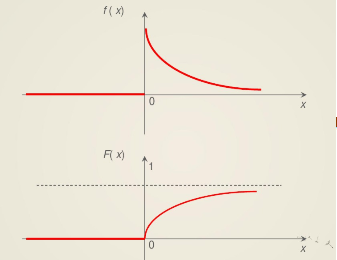=====
回到本题，
指数分布的分布函数是
分布函数为F(x)=1−e−λx$F\left(x\right)=1-{e}^{-\lambda x}$$F(x)=1-e^{-\lambda x}$
其意义是指在x时间内事件发生的概率，其等于t时间内出现一个以上的概率，等于P{X>0,N(t)}=1−e−λt$其意义是指在x时间内事件发生的概率，其等于t时间内出现一个以上的概率，等于P\left\{X>0,N\left(t\right)\right\}=1-{e}^{-\lambda t}$${\color{Red} {其意义是指在 x时间内事件发生的概率}}，其等于t 时间内出现一个以上的概率，等于P\{ X>0,N(t) \}=1-e^{-\lambda t}$。

本题求系统正常工作的时间的概率分布，
已知一个元件正常工作的概率分布（t时间内发生故障的概率）F(t)=1−e−λt$F\left(t\right)=1-{e}^{-\lambda t}$$F(t)=1-e^{-\lambda t}$
系统正常工作，要求三个元件都不发生故障，F(t)是发生故障的概率，不发生故障的概率是1-F(t), 如果是三个F(t)相乘，等于三个同时发生故障的概率，一个都不发生故障，应该是三个(1-F(t))相乘，系统要求求三个都不发生故障的分布函数，就是1-三个（1-F(t))相乘。

所以
FT(t)=1−（1−F1(t)）∗（1−F2(t)）∗（1−F3(t)）${F}_{T}\left(t\right)=1-（1-{F}_{1}\left(t\right)）\ast （1-{F}_{2}\left(t\right)）\ast （1-{F}_{3}\left(t\right)）$$F_{T}(t) = 1-（1-F_{1}(t)）*（1-F_{2}(t)）*（1-F_{3}(t)）$
=1−(e−λt)3$=1-\left({e}^{-\lambda t}{\right)}^{3}$$=1 - ( e^{-\lambda t}) ^3$              (1)

如果是三个F_{T}(t)直接相乘，得到的结果是
FT1(t)=F1(t)∗F2(t)∗F3(t)${F}_{T1}\left(t\right)={F}_{1}\left(t\right)\ast {F}_{2}\left(t\right)\ast {F}_{3}\left(t\right)$$F_{T1}(t) = F_{1}(t)*F_{2}(t)*F_{3}(t)$
=(1−e−λt)3$=\left(1-{e}^{-\lambda t}{\right)}^{3}$$= ( 1-e^{-\lambda t}) ^3$
=1−3e−λt+3e−2λt−e3λt$1-3{e}^{-\lambda t}+3{e}^{-2\lambda t}-{e}^{3\lambda t}$$1-3e^{-\lambda t}+3e^{-2\lambda t} -e^{3\lambda t}$ = 1−3e−λt(1−e−λt)−e3λt$1-3{e}^{-\lambda t}\left(1-{e}^{-\lambda t}\right)-{e}^{3\lambda t}$$1-3e^{-\lambda t}(1-e^{-\lambda t})-e^{3\lambda t}$    (2)
其中，(1−e−λt)$\left(1-{e}^{-\lambda t}\right)$$(1-e^{-\lambda t})$ 肯定是大于0 的，所以(2)式的整体值小于(1)式，
FT1(t)${F}_{T1}\left(t\right)$$F_{T1}(t)$ 小于 FT(t)${F}_{T}\left(t\right)$$F_{T}(t)$ ， 而F(t)的含义是在t时间前系统发生故障的概率， 而实际上要求三个元件同时不发生故障系统才算不发生故障， 所以F(t)应该取大才对。

还有一个公式
在书上P82(2).3${P}_{82}\left(2\right).3$$P_{82}(2).3$：
设X,Y相互独立，分布函数分别为F_{X}(x)和F_{Y}(y)，M=max(X.Y),  N=min(X,Y)，则
FM(z)=FX(z)∗FY(z),FN(z)=1−[1−FX(z)][1−FY(z)]${F}_{M}\left(z\right)={F}_{X}\left(z\right)\ast {F}_{Y}\left(z\right),{F}_{N}\left(z\right)=1-\left[1-{F}_{X}\left(z\right)\right]\left[1-{F}_{Y}\left(z\right)\right]$$F_{M}(z)=F_{X}(z)*F_{Y}(z) , F_{N}(z)=1-[1-F_{X}(z)][1-F_{Y}(z)]$

本题中 T=min(X1, X2, X3)，故 G(t)=1−[1−F(t)]3$G\left(t\right)=1-\left[1-F\left(t\right){\right]}^{3}$$G(t)=1-[1-F(t)]^3$

【例四】

设随机变量(X,Y)在矩形区域G=((x,y)|0⩽x⩽2,0⩽y⩽1)$G=\left(\left(x,y\right)|0⩽x⩽2,0⩽y⩽1\right)$$G=((x,y)|0\leqslant x\leqslant 2,0\leqslant y\leqslant 1)$上服从均匀分布，试求Z=XY的密度函数。

【 解 】

需要理解的是：二维随机变量均匀分布的概率密度函数=1分布区域的面积$二维随机变量均匀分布的概率密度函数=\frac{1}{分布区域的面积}$${\color{Red} {二维随机变量均匀分布的概率密度函数 = \frac{1}{分布区域的面积} }}$

区域G的面积=x的长度乘以y的宽度=(2-0) x (1-0)=2*1=2

X和Y的均匀分布的概率密度函数 = 1分布区域的面积=12$\frac{1}{分布区域的面积}=\frac{1}{2}$$\frac{1}{分布区域的面积}=\frac{1}{2}$

区域G的面积=x的长度y的宽度=(2-0)(1-0)
均匀分布的密度函数 = 1区域G的面积=12∗1=12$\frac{1}{区域G的面积}=\frac{1}{2\ast 1}=\frac{1}{2}$$\frac{1}{区域G的面积} = \frac{1}{2*1} = \frac{1}{2}$

对于 Z=X*Y的密度函数与Z=X+Y的密度函数有所不同。
见P82.(2)

Z=X+Y型的密度函数为，fZ(z)=∫∞−∞f(x,z−x)dx$Z=X+Y型的密度函数为，{f}_{Z}\left(z\right)={\int }_{-\mathrm{\infty }}^{\mathrm{\infty }}f\left(x,z-x\right)dx$${\color{Red} {Z=X+Y型的密度函数为，f_{Z}(z)=\int_{-\infty}^{\infty}f(x,z-x)dx}}$

Z=X/Y型的密度函数为，fY/X(z)=∫∞−∞|x|f(x,zx)dx$Z=X/Y型的密度函数为，{f}_{Y/X}\left(z\right)={\int }_{-\mathrm{\infty }}^{\mathrm{\infty }}|x|f\left(x,zx\right)dx$${\color{Red} {Z=X/Y型的密度函数为，f_{Y/X}(z)=\int_{-\infty}^{\infty}|x|f(x,zx)dx}}$

Z=XY型的密度函数为，fYX(z)=∫∞−∞1|x|f(x,zx)dx$Z=XY型的密度函数为，{f}_{YX}\left(z\right)={\int }_{-\mathrm{\infty }}^{\mathrm{\infty }}\frac{1}{|x|}f\left(x,\frac{z}{x}\right)dx$${\color{Red} {Z=XY型的密度函数为，f_{YX}(z)=\int_{-\infty}^{\infty}\frac{1}{|x|}f(x,\frac{z}{x})dx}}$

对于本题，要求Z=XY的密度函数
Z=XY型的密度函数为，fYX(z)=∫∞−∞1|x|f(x,zx)dx$Z=XY型的密度函数为，{f}_{YX}\left(z\right)={\int }_{-\mathrm{\infty }}^{\mathrm{\infty }}\frac{1}{|x|}f\left(x,\frac{z}{x}\right)dx$$Z=XY型的密度函数为，f_{YX}(z)=\int_{-\infty}^{\infty}\frac{1}{|x|}f(x,\frac{z}{x})dx$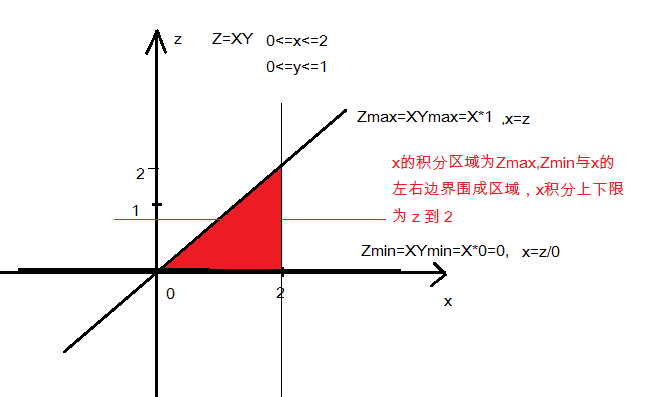如图所示，
Zmax = X*Ymax = X*1 =X, ==> 直线边界：x=z
Zmin = X*Ymin = X*0 =0 , ==> 直线边界：z=0
X的左右边界为 0 到 2
所以积分区域就是Zmax, Zmin和X的左右边界围成的区块

对x进行积分，x的左边界为Zmax直线，即x=z，右边界为x=2垂直直线。
当 0⩽z<2时，fXY(z)=∫∞−∞1|x|f(x,zx)dx=∫2z1|x|∗12dx$0⩽z<2时，{f}_{XY}\left(z\right)={\int }_{-\mathrm{\infty }}^{\mathrm{\infty }}\frac{1}{|x|}f\left(x,\frac{z}{x}\right)dx={\int }_{z}^{2}\frac{1}{|x|}\ast \frac{1}{2}dx<$